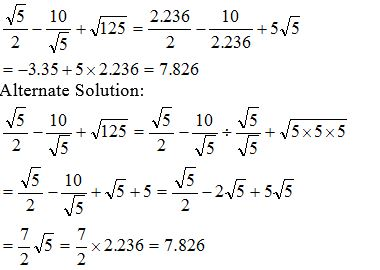# Sample Aptitude Questions of Newgen

1. In an election there were only two candidates. One of the candidates secured 40% of votes and is defeated by the other candidate by 298 votes. The total number of votes polled is
1. 1490
2. 1500
3. 745
4. 1460
Let x be the total no of votes
Therefore, 40% × x + 298 = 60% × x => 20% × x = 298 => x = 1490
Alternatively we can also say if one candidate got 40 % votes then other got 60 % votes , Therefore difference is 20 % votes
20 % of votes = 298
Therefore 100 % = 1490.
2. 122 + 162 + 222 + 2 × 12 × 16 - 2 × 22 × 16 - 2 × 12 × 22 = ?
1. 50
2. 36
3. 6
4. 18
As per formula, ( a + b - c )2 = a2 + b2 + c2 + 2ab – 2bc – 2ac
Therefore, ( 12 + 16 - 22 )2 = 36
1. The roots of the equation 4x2 + 9x + 4 = 0 are
1. Reciprocals
2. Positive
3. Equal
4. Imaginary
In standard equation ax2 + bx + c, whenever a = c, the roots are reciprocal of each other.
2. If |2x + 3| > 8, then which of the following is true?
1. -5.5 > x > 2.5
2. 2.5 > x > -5.5
3. 5.5 > x > -2.5
4. -5.5 > x > -2.5
Either ( 2x + 3 ) > 8 or –( 2x + 3 ) > 8, so we get 2x > 5 or -2x > 11 i.e. x > 2.5 or x < -5.5 - 5.5 > x > 2.5
3. In a container there is 90 ltrs of mixture of milk and water in the ratio 11:4. What part of the mixture should be replaced with same quantity of water, so that the ratio after replacement becomes 11:7?
1. 5/6
2. 3/11
3. 1/6
4. 1/3
In 90 ltrs., the ratio of milk and water is 11:4 i.e. M = 66 ltr & W = 24 ltr
After the replacement, the ratio becomes 11:7, i.e. M = 55 ltr & W = 35 ltr
Since qty. of milk is reduced to1/6th , therefore the overall removal is also 1/6th
4. Ram can do a certain work in 16 days and Shyam can do the same work in 10 days. If they start the work together, then how many days early can Shyam quit, if the work is to be done in 8 days?
1. 3 days
2. 5 days
3. 6 days
4. 2 days
Ram does (1/16) × 8 = 1/2 work in 8 days, so the other half has to be done by Shyam, for which he will need 10/2 = 5 days. So he can quit ( 8 - 5 ) = 3 days early.
5. A jar contains 10 red marbles and 30 green ones. How many red marbles must be added to the jar so that 60% of the marbles will be red?
1. 25
2. 30
3. 35
4. 40
Let 'x' red marbles will be added to the jar Then, according to the conditions,
( 10 + x ) = 60 / 100 × ( 40 + x ) ==> x = 35
6. If a number multiplied by 25% of itself gives a number which is 200% more than the number, then the number is
1. 12
2. 16
3. 20
4. 24
Let the no. be x.
Then x * ( 25/100 * x ) = x + 200/100 * x
==> x = 12
7. The square root of ( 7 + √35 ) ( 7 - √35 ) is
1. √14
2. √17
3. 14
4. 3√5